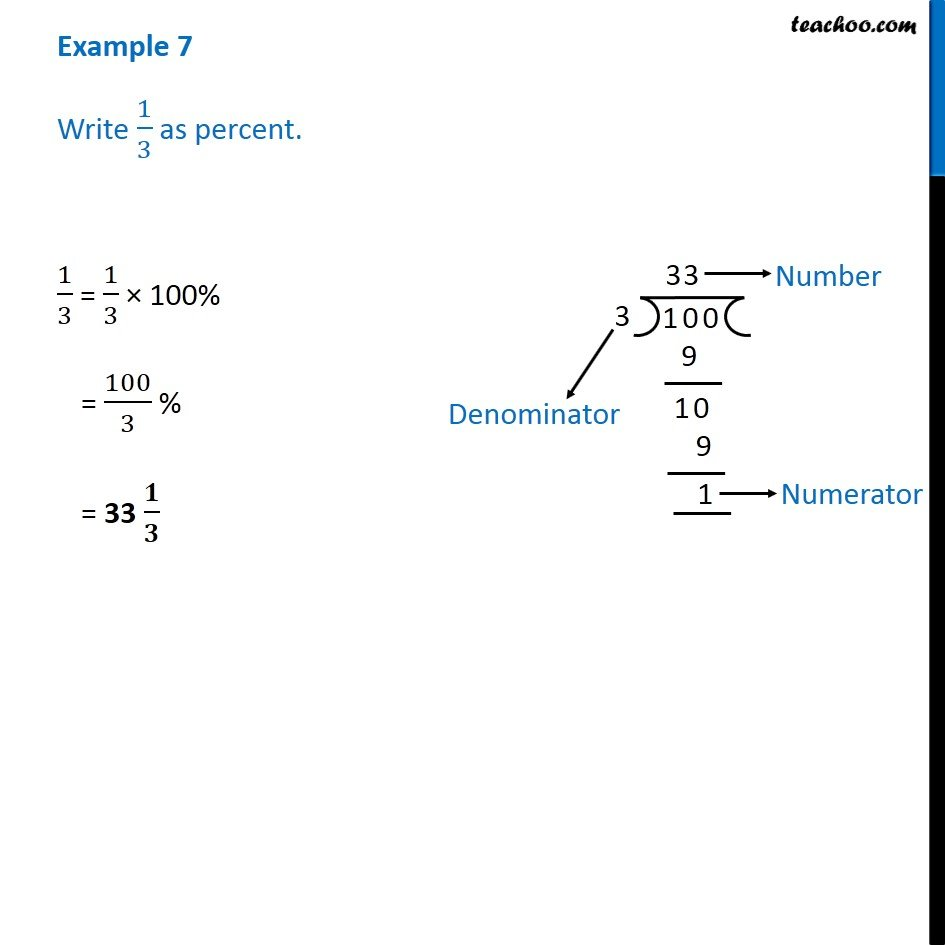1. Chapter 8 Class 7 Comparing Quantities
2. Serial order wise
3. Examples

Transcript

Example 7 - Chapter 8 Class 7 Comparing Quantities - NCERT Solutions Write 1/3 as percent. To write fraction as percentage, we multiply number by 100% 1/3 = 1/3 × 100% = 100/3 % = 33 𝟏/𝟑 % Division of 100 by 3 is given in the figure

Examples